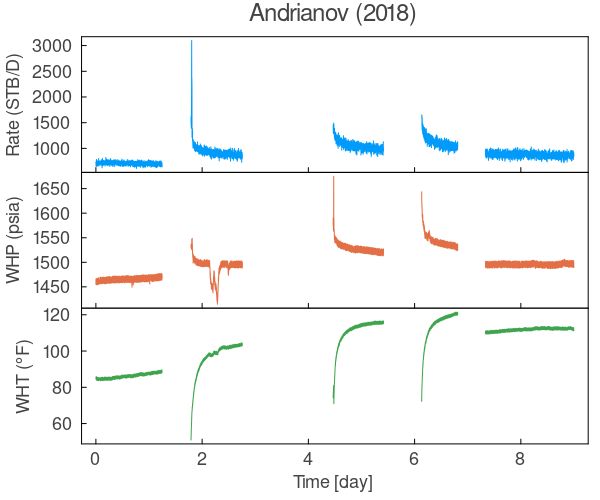# Plots.jl: Creating stacked subplots with common x-axis and no margins (padding) in between

Hello!

I would like to know if it is possible to create a plot with stacked subplots sharing a common x-axis.
I’m looking to have no margins, gaps, spaces, or paddings in between the subplots.

Is it possible to do it in Plots.jl? I know that I can manually set the margins in between, but I’d like to know if there is a way to do it automatically.

Here is an example of what I’m trying to achieve.Appreciate the help.

Okay, so I have coded up a plot which should generate the example I’m trying to achieve.
I don’t know if this is the best way, but it does get the job done so far.

I hope there is a better way than this.

``````function vstackplot(
plts...;
top_margin=:match, bottom_margin=:match,
kw...
)
n   = length(plts)
plt = plot(plts..., link=:x, layout=(n, 1); kw...)

Plots.prepare_output(plt) # to get Plots.jl to calculate bounding boxes and plotting areas

margin = 0.0mm
for i in 1:n
sp = plt.subplots[i]

bb_top = Plots.top(sp.bbox)
bb_bot = Plots.bottom(sp.bbox)

pa_top = Plots.top(sp.plotarea)
pa_bot = Plots.bottom(sp.plotarea)

mg_top = pa_top - bb_top
mg_bot = bb_bot - pa_bot

if i != 1
margin -= mg_top
end

if i != n
margin -= mg_bot
end
end

w, h    = Plots.get_size(plt)
margin /= 2*(n - 1)
margin /= w/500 # I have no idea why this works but it does

for i in 1:n
sp = plt.subplots[i]

if i != 1
plot!(sp, top_margin=margin)
end

if i != n
plot!(sp, bottom_margin=margin)
plot!(sp, x_foreground_color_text=:white)
end
end

plot!(plt.subplots; top_margin)
plot!(plt.subplots[end]; bottom_margin)

return plt
end
``````